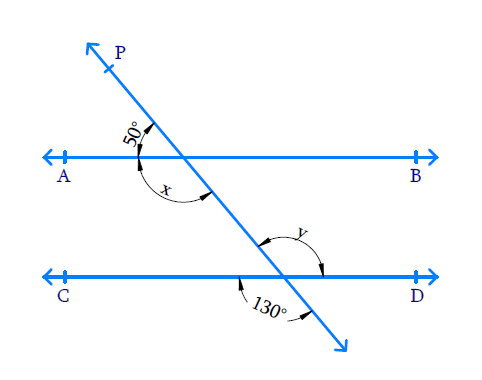# Ex.6.2 Q1 Lines and Angles Solution - NCERT Maths Class 9

Go back to  'Ex.6.2'

## Question

In the given figure, find the values of $$x$$ and $$y$$ and then show that $$AB ‖ CD$$.Video Solution
Lines And Angles
Ex 6.2 | Question 1

## Text Solution

Reasoning:

• When two lines intersect, vertically opposite angles formed are equal.
• Also, when a ray intersects a line sum of adjacent angles formed is $$180^ {\circ}$$.
• If a transversal intersects two lines such that a pair of alternate angles is equal, then the two lines are parallel to each other.

Steps:

Line $$CD$$ is intersected with line $$P$$, hence the vertically opposite angles so formed are equal. $$y = 130^ {\circ}.$$

Similarly, line $$AB$$ is intersected by line $$P$$ hence the sum of adjacent angles formed is $$180^ {\circ}.$$

\begin{align} x + 50 ^ { \circ } & = 180 ^ { \circ } \\ x & = 180 ^ { \circ } - 50 ^ { \circ } \\ & = 130 ^ { \circ } \end{align}

We know that, if a transversal intersects two lines such that a pair of alternate interior angles is equal, then the two lines are parallel. Here we can see that the pair of alternate angles formed when lines $$AB$$ and $$CD$$ are intersected by transversal $$P$$ are equal. Therefore, $$x = y = 130^ {\circ}.$$ So we can say the two lines $$AB$$ and $$CD$$ are parallel. Hence $$AB ‖ CD$$ is proved.

Video Solution
Lines And Angles
Ex 6.2 | Question 1

Learn from the best math teachers and top your exams

• Live one on one classroom and doubt clearing
• Practice worksheets in and after class for conceptual clarity
• Personalized curriculum to keep up with school